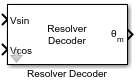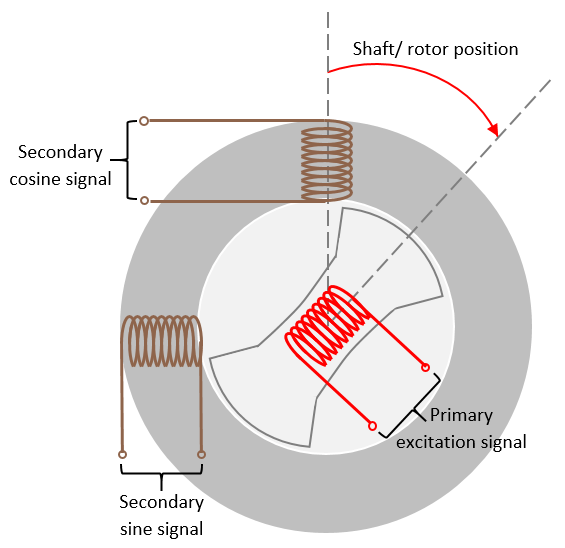# Resolver Decoder

Compute motor mechanical position and speed as well as sine and cosine values of motor electrical position

Since R2020a

•Libraries:
Motor Control Blockset / Sensor Decoders

## Description

The Resolver Decoder block computes the following for a resolver connected to a motor shaft:

• Mechanical angular position of motor

• Sine and cosine of electrical angular position of motor

• Mechanical speed of motor

A resolver uses a primary excitation input signal to generate the modulated secondary sine and cosine waveforms, which is then sampled by the ADC. The resolver utilizes one winding to two winding transformations. The sine and cosine modulation occurs in the secondary windings because of the design and construction of these windings, which places them at positions that are 90 degrees apart.For more details about the algorithm used by the block, see Algorithm.

## Ports

### Input

expand all

Secondary sine waveform output from the resolver that is sampled. When using sinusoidal excitation, this signal should be normalized within the range of [-1, 1] and centred at 0.

Data Types: `single` | `double` | `fixed point`

Secondary cosine waveform output from the resolver that is sampled. When using sinusoidal excitation, this signal should be normalized within the range of [-1, 1] and centred at 0.

Data Types: `single` | `double` | `fixed point`

### Output

expand all

Mechanical angular position of the resolver (and the motor) in either degrees, radians, or per-unit.

Data Types: `single` | `double` | `fixed point`

Sine of electrical angular position of the motor.

Data Types: `single` | `double` | `fixed point`

Cosine of electrical angular position of the motor.

Data Types: `single` | `double` | `fixed point`

Mechanical speed of the motor in either degrees/sec, radians/sec, RPM, or per-unit.

Data Types: `single` | `double` | `fixed point`

## Parameters

expand all

The output of the Resolver Decoder block:

• Mechanical position — Enable the block to compute motor mechanical position.

• Sine and Cosine electrical position — Enable the block to compute sine and cosine values of motor electrical position.

• Mechanical speed — Enable the block to compute the mechanical speed of the motor.

### Input Parameters

The number of pole pairs available in the resolver.

The resolver excitation method used to obtain secondary sine and cosine waveforms.

When using square pulse excitation method, the block normalizes the secondary sine and cosine inputs only if you select this parameter.

#### Dependencies

To enable this parameter, set Type of excitation method to `Square Pulse Excitation`.

The fixed time interval (in seconds) between every two consecutive instances of block execution.

#### Dependencies

To enable this parameter, set Block Output to `Mechanical speed`.

### Inputs for Sine and Cosine electrical position

The number of pole pairs available in the motor.

#### Dependencies

To enable this parameter, set Block Output to `Sine and Cosine electrical position`.

The offset between resolver mechanical position and motor mechanical position (in radians).

#### Dependencies

To enable this parameter, set Block Output to `Sine and Cosine electrical position`.

Select the rounding mode for fixed-point operations used when computing sine and cosine of motor electrical position. You can select:

• Simplest — Generates compact rounding code with faster computation. However, with time, it may add bias in sine and cosine electrical position output.

• Zero — Ensures that the rounding code does not add any bias in the sine and cosine electrical position output. However, this results in slower computation.

#### Dependencies

To enable this parameter, set Block Output to `Sine and Cosine electrical position`.

### Inputs for sinusoidal excitation method

The phase delay that the block must add to the `Sin` and `Cos` input port signals.

#### Dependencies

To enable this parameter, set Type of excitation method to `Sinusoidal Excitation`.

Number of samples available in one cycle of the `Sin` and `Cos` input port signals.

#### Dependencies

To enable this parameter, set Type of excitation method to `Sinusoidal Excitation`.

### Output Parameters

The unit of the mechanical position output of the block.

#### Dependencies

To enable this parameter, set Block Output to `Mechanical position`.

The unit of the mechanical speed output of the block.

#### Dependencies

To enable this parameter, set Block Output to `Mechanical speed`.

The rated speed of the motor (in RPM).

#### Dependencies

To enable this parameter, set Speed unit to `Per-Unit` and Block Output to `Mechanical speed`.

## Algorithms

expand all

The block uses the correctly sampled and normalized version of secondary sine and cosine waveforms to demodulate the sine and cosine signals using which it determines the electrical position of the resolver. It converts this position into its mechanical equivalent according to the number of pole pairs available in the resolver. The resulting value indicates the mechanical position of the motor.

The block also uses the demodulated sine and cosine signals (sine and cosine of resolver electrical angle) to compute the motor speed as well as the sine and cosine values of the motor electrical position.

## Version History

Introduced in R2020a